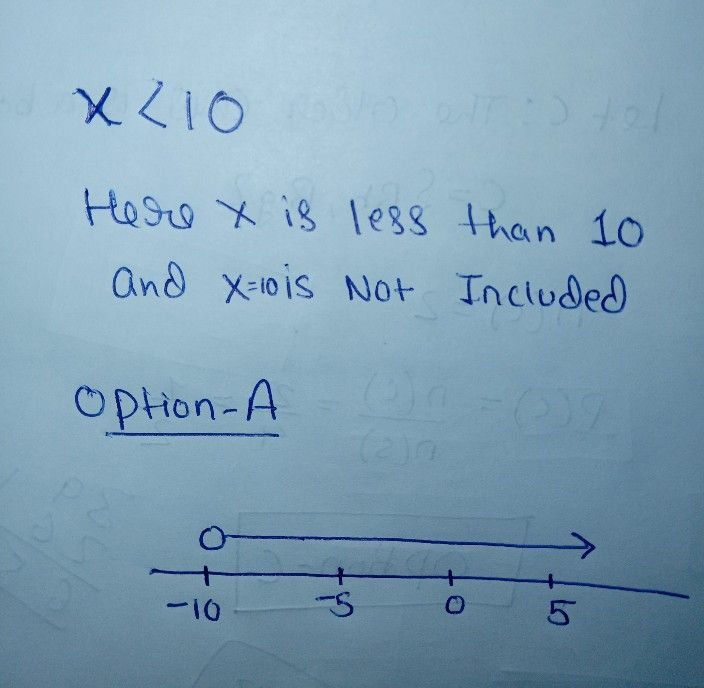Symbol
Problem$7.0$ Graph the following inequality on the number line. $x<10$ $○$ $-10$ $=5$ 5n $10$ $○$ $10$ $1.5$ $2.0$ $2.5$ $3.0$ $-10$ $-5$ 5. $10$ $○$ $-10$ $-5$ 10
7th-9th grade
Algebra
SolutionQanda teacher - kavita❤️